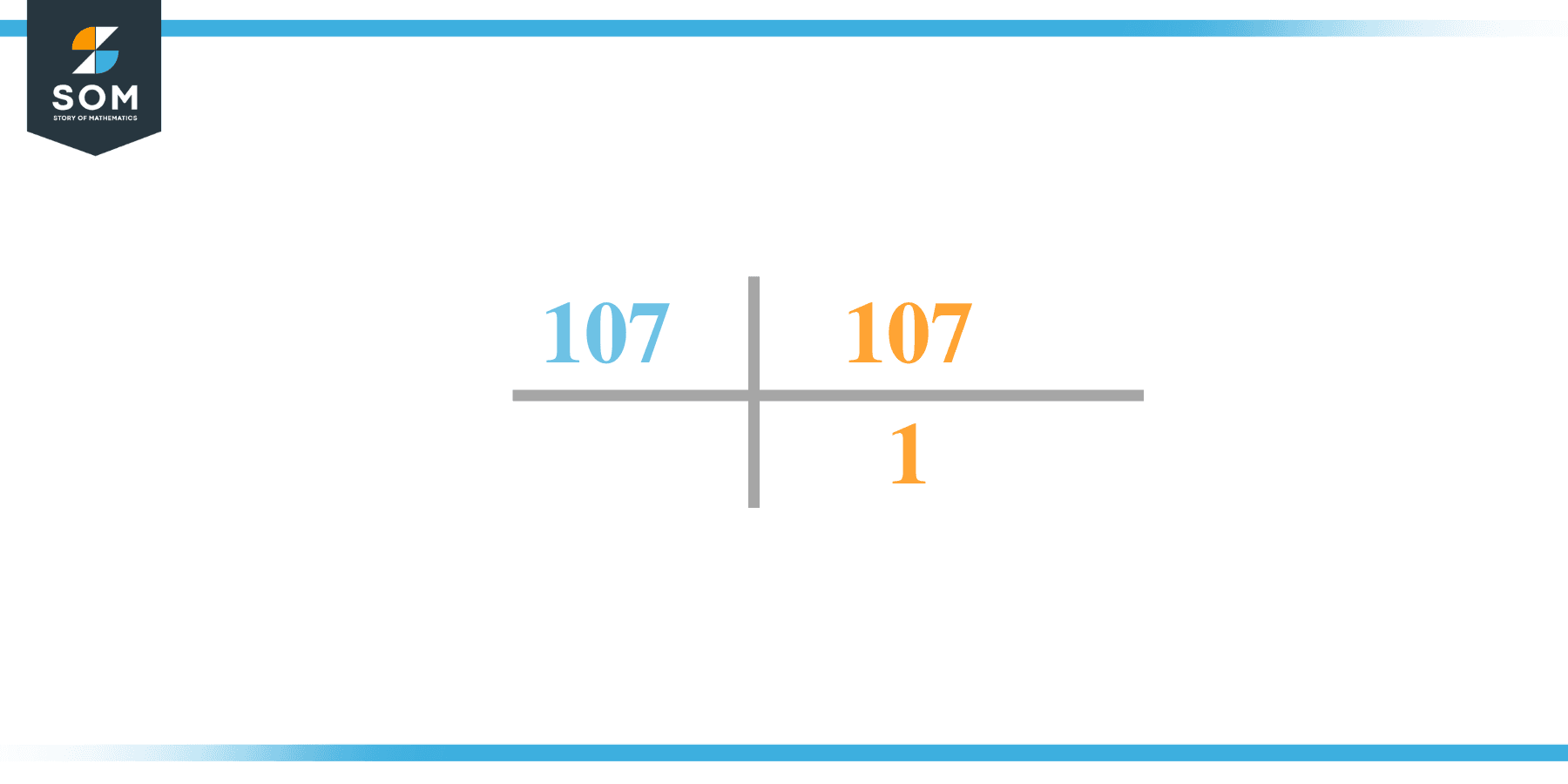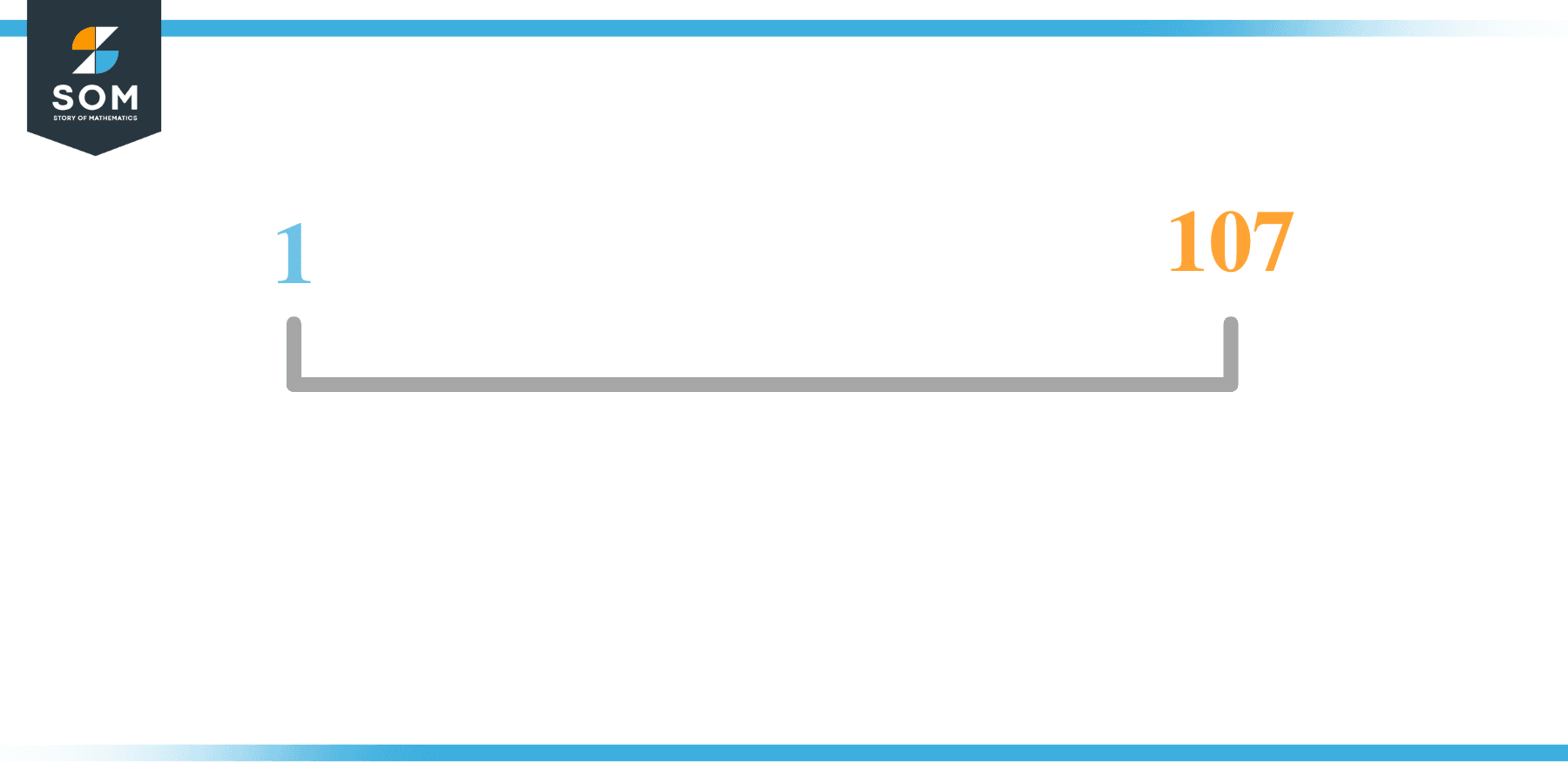# Factors of 107: Prime Factorization, Methods, and Examples

The factors of 107 are numbers that give leave zero as a remainder when 107 is divided from them. In other words, the factors of 107 are the numbers that produce 107 as the product when multiplied together.The number 107 is a prime number so it only consists of two factors; the smallest factor 1 and the number itself.

### Factors of 107

Here are the factors of number 107.

Factors of 107: 1, 107

### Negative Factors of 107

The negative factors of 107 are similar to its positive factors, just with a negative sign.

Negative Factors of 107: -1, and -107 \]

### Prime Factorization of 107

The prime factorization of 107 is the way of expressing prime factors of a number in the form of a product.

Prime Factorization: 1x 107

In this article, we will learn about the factors of 107 and how to find them using various techniques such as upside-down division, prime factorization, and factor tree.

## What Are the Factors of 107?

The factors of 107 are 1 and 107. All of these numbers are the factors as they do not leave any remainder when divided by 107.

The factors of 107 are classified as prime numbers and composite numbers. The prime factors of the number 107 can be determined using the technique of prime factorization.

## How To Find the Factors of 107?

You can find the factors of 107 by using the rules of divisibility. The rule of divisibility states that any number when divided by any other natural number then it is said to be divisible by the number if the quotient is the whole number and the resulting remainder is zero.

To find the factors of 107, create a list containing the numbers that are exactly divisible by 107 with zero remainders. One important thing to note is that 1 and 107 are the 107’s factors as every natural number has 1 and the number itself as its factor.

1 is also called the universal factor of every number. The factors of 107 are determined as follows:

$\dfrac{107}{1} = 107$

$\dfrac{107}{107} = 1$

Therefore, 1 and 107 are the factors of 107.

### Total Number of Factors of 107

For 107 there are 2 positive factors and 2 negative ones. So in total, there are 4 factors of 107.

To find the total number of factors of the given number, follow the procedure mentioned below:

1. Find the factorization of the given number.
2. Demonstrate the prime factorization of the number in the form of exponent form.
3. Add 1 to each of the exponents of the prime factor.
4. Now, multiply the resulting exponents together. This obtained product is equivalent to the total number of factors of the given number.

By following this procedure the total number of factors of 107 is given as:

Factorization of 107 is 1 x 107.

The exponent of 1 and 107 is 1.

Adding 1 to each and multiplying them together results in m.

Therefore, the total number of factors of 107 is 4 where 2 are positive factors and 2 are negative factors.

### Important Notes

Here are some important points that must be considered while finding the factors of any given number:

• The factor of any given number must be a whole number.
• The factors of the number cannot be in the form of decimals or fractions.
• Factors can be positive as well as negative.
• Negative factors are the additive inverse of the positive factors of a given number.
• The factor of a number cannot be greater than that number.
• Every even number has 2 as its prime factor which is the smallest prime factor.

## Factors of 107 by Prime FactorizationThe number 107 is a prime number. Prime factorization is a useful technique for finding the number’s prime factors and expressing the number as the product of its prime factors.

Before finding the factors of 107 using prime factorization, let us find out what prime factors are. Prime factors are the factors of any given number that are only divisible by 1 and themselves.

To start the prime factorization of 107, start dividing by its smallest prime factor. First, determine that the given number is either even or odd. If it is an even number, then 2 will be the smallest prime factor.

Continue splitting the quotient obtained until 1 is received as the quotient. The prime factorization of 107 can be expressed as:

107= 1 x 107

## Factors of 107 in PairsThe factor pairs are the duplet of numbers that when multiplied together result in the factorized number. Depending upon the total number of factors of the given numbers, factor pairs can be more than one.

For 107, the factor pairs can be found as:

1 x 107 = 107

The possible factor pair of 107 is given as (1, 107).

All these numbers in pairs, when multiplied, give 107 as the product.

The negative factor pairs of 107 are given as:

-1 x -107 = 107

It is important to note that in negative factor pairs, the minus sign has been multiplied by the minus sign due to which the resulting product is the original positive number. Therefore, -1 and -107 are called negative factors of 107.

The list of all the factors of 107 including positive as well as negative numbers is given below.

Factor list of 107: 1, -1, 107, -107

## Factors of 107 Solved Examples

To better understand the concept of factors, let’s solve some examples.

### Example 1

How many factors of 107 are there?

### Solution

The total number of Factors of 107 is 2.

Factors of 107 are 1 and 107.

### Example 2

Find the sum of the factors of 107.

### Solution

The factors of 107 are 1 and 107. Their sum is given below:

Sum = 1 + 107 = 108